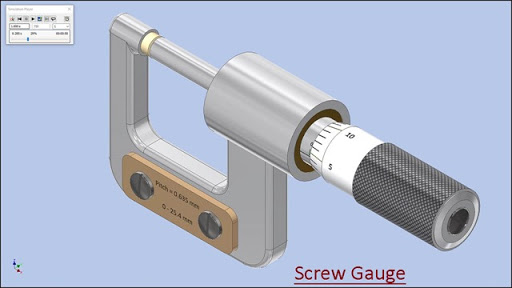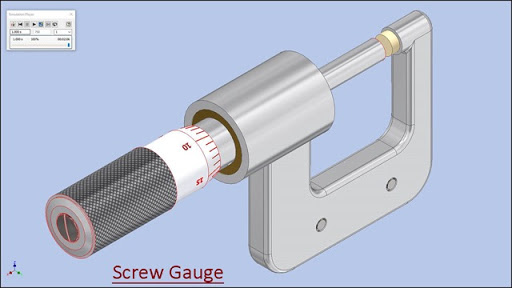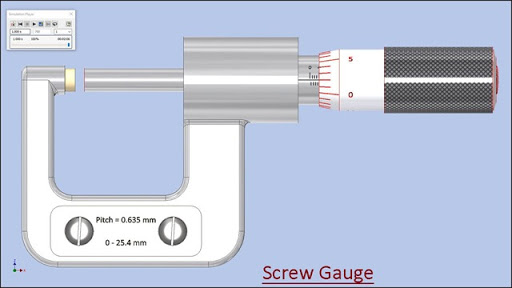Friday, September 28, 2018

Modelling of 'Screw Gauge' with Dynamic Simulation (Volume-1) Autodesk Inventor 2018 TutorialSerial No. 305-A.

Modelling of 'Screw Gauge' with Dynamic Simulation (Volume-1) Autodesk Inventor 2018 Tutorial

(Rest of the model is completed in Volume-2)Click the following link to get the model file: - https://autode.sk/2DLeKjg

Procedure of reading of Screw Gauge

Total number of divisions imprinted on Circular scale (in one round) = 25

Total number of divisions imprinted on Linear scale = 40

Pitch of Linear scale = total length of Linear scale/total number of divisions imprinted on Linear scale
= 25.4mm/40 = 0.635mm

Least count of Circular scale = Pitch of Linear scale/total number of divisions imprinted on Circular scale
= 0.635mm/25 = 0.0254mm

Reading of Linear scale = Pitch of Linear scale x Number of divisions opened on Linear scale
= 0.635mm x 4 = 2.54mm

Reading of Circular scale = Least count of Circular scale x Number of divisions
ahead of central line of Linear scale
= 0.0254mm x 2 = 0.0508mm